# Toroidal harmonics

Functions of the points on a torus that arise when solving the Laplace equation by the method of separation of variables (cf. Separation of variables, method of) in toroidal coordinates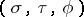. A harmonic function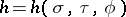, which is a solution of the Laplace equation, can be written as a series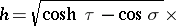(*)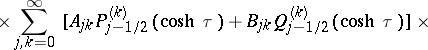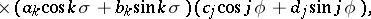where the,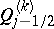are the associated Legendre functions with half-integer index. By setting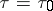one obtains a toroidal harmonic or a surface toroidal harmonic, this in contrast with the expression (*) which, as a function of the three variables, is sometimes called a spatial toroidal harmonic.

The series (*) is used in the solution of boundary value problems in toroidal coordinates, taking into account the expansion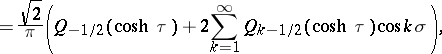where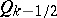is the Legendre function of the second kind.